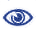# The Prague Stringology Conference 2006

Reachability on Suffix Tree Graphs

 Abstract: We analyze the complexity of graph reachability queries on ST-graphs, defined as directed acyclic graphs (DAGs) obtained by merging the suffix tree of a given string and its suffix links. Using a simplified reachability labeling algorithm presented by Agrawal et al. (1989), we show that for a random string of length n, its ST-graph can be preprocessed in O(n log n) expected time and space to answer reachability queries in O(log n) time. Furthermore, we present a series of strings that require Θ(n n0.5) time and space to answer reachability queries in O(log n) time for the same algorithm. Exhaustive computational calculations for strings of length n ≤ 33 have revealed that the same strings are also the worst case instances of the algorithm. We therefore conjecture that reachability queries can be answered in O(log n) time with a worst case time and space preprocessing complexity of Θ(n n0.5).

 Download paper:PostScript PDF BibTeX reference
 Download presentation: